# Arithmetic space

number space, coordinate space, real-space

A Cartesian power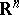of the set of real numbers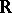having the structure of a linear topological space. The addition operation is here defined by the formula: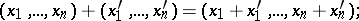while multiplication by a number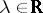is defined by the formula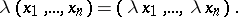The topology inis the topology of the direct product ofcopies of; its base is formed by open-dimensional parallelepipeda:where the numbers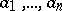and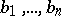are given.

The real-spaceis also a normed space with respect to the normwhere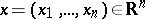, and is a Euclidean space with respect to the scalar product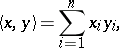where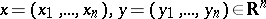.

How to Cite This Entry:
Arithmetic space. I.V. Dolgachev (originator), Encyclopedia of Mathematics. URL: http://www.encyclopediaofmath.org/index.php?title=Arithmetic_space&oldid=13139
This text originally appeared in Encyclopedia of Mathematics - ISBN 1402006098